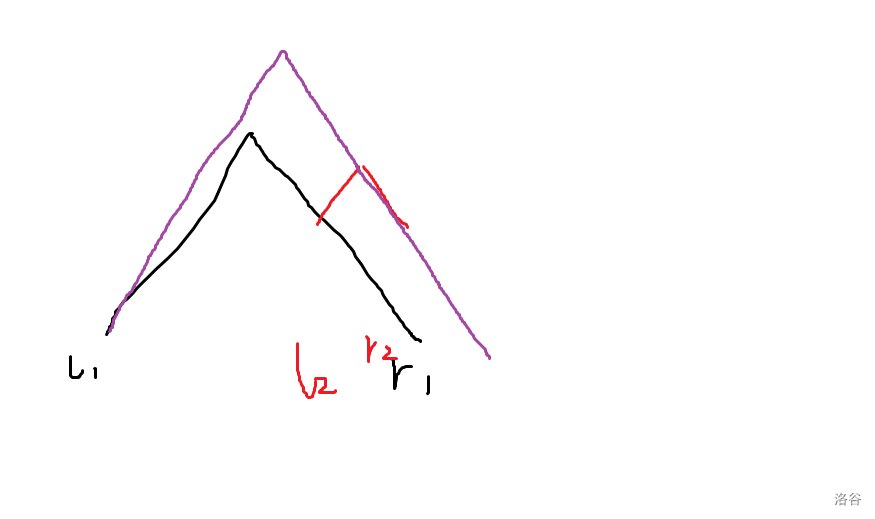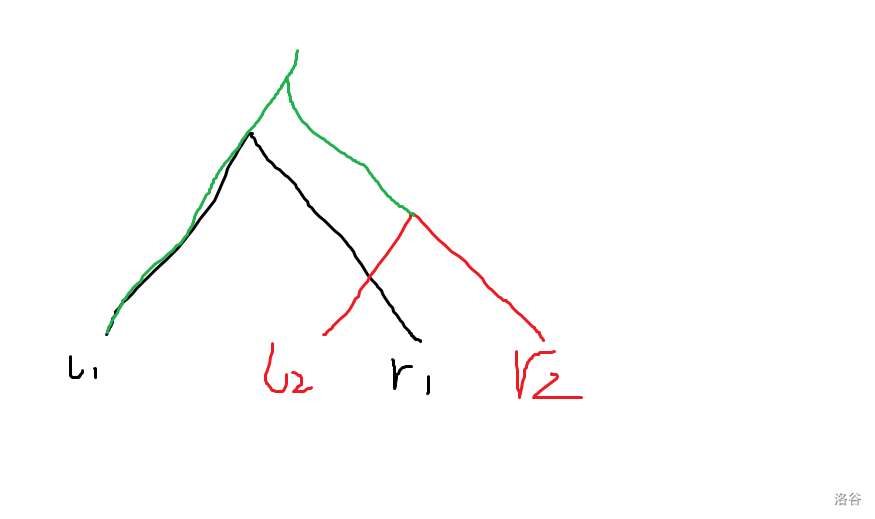# Doubeecat's Blog

“即便前路混沌，同她走过，才算人间。”

0%

# 2021.5.6

## CF1515F

• 如果$a_p \geq x$ 那这里我们可以直接让 $p$ 和它的父亲连起来
• 如果$a_p < x$，我们可以把 $p$ 去掉，然后在剩下的处理。根据鸽巢原理，肯定是存在一个 $u$ 使 $a_u > x$，那么如果这里 $x \times (n-1) < \sum a_i$ 就无法构造，反之则一定存在一种构造方案。

# 2021.5.7-2021.5.8

## CFgym102331B

• 若 $x_3 = 0$：
如果此时 $x_1 \operatorname{xor} x_2 = 1$，那么低位来看肯定有 $a_i \operatorname{xor} x_j \geq x$。所以这里 $x_1,x_2$ 就有两种情况，分别讨论掉就可以。直接把整颗子树加进来，接下来就朝着 $x_1 \operatorname{xor} x_2 \operatorname{xor} x_3$ 的方向走，代码长这样：
• 若 $x_3 = 1$：
那你这里做什么都不能保证是一定包含的，直接走到 $x_3 \operatorname{xor} 1$ 即可。

trie 写的比较少，这里贴代码，不会的可以问我，被这个题搞了两天

# 2020.5.9

## P7595 猜树

Sub 4：先用询问 2 求出每个节点的深度，然后暴力枚举它的儿子询问距离。若二元组 (u,v) 距离为 1，且 $dep_v = dep_u+1$, 则 $v$ 在 $u$ 的子树，大力判断一下就行了，时间复杂度是 $O(n)$ 的。

Sub 5：完全二叉树最多只有 logn 层，考虑逐层遍历。先用询问 2 求出每个节点的深度。

# 2020.5.17

## P7594 「EZEC-8」Clear Up